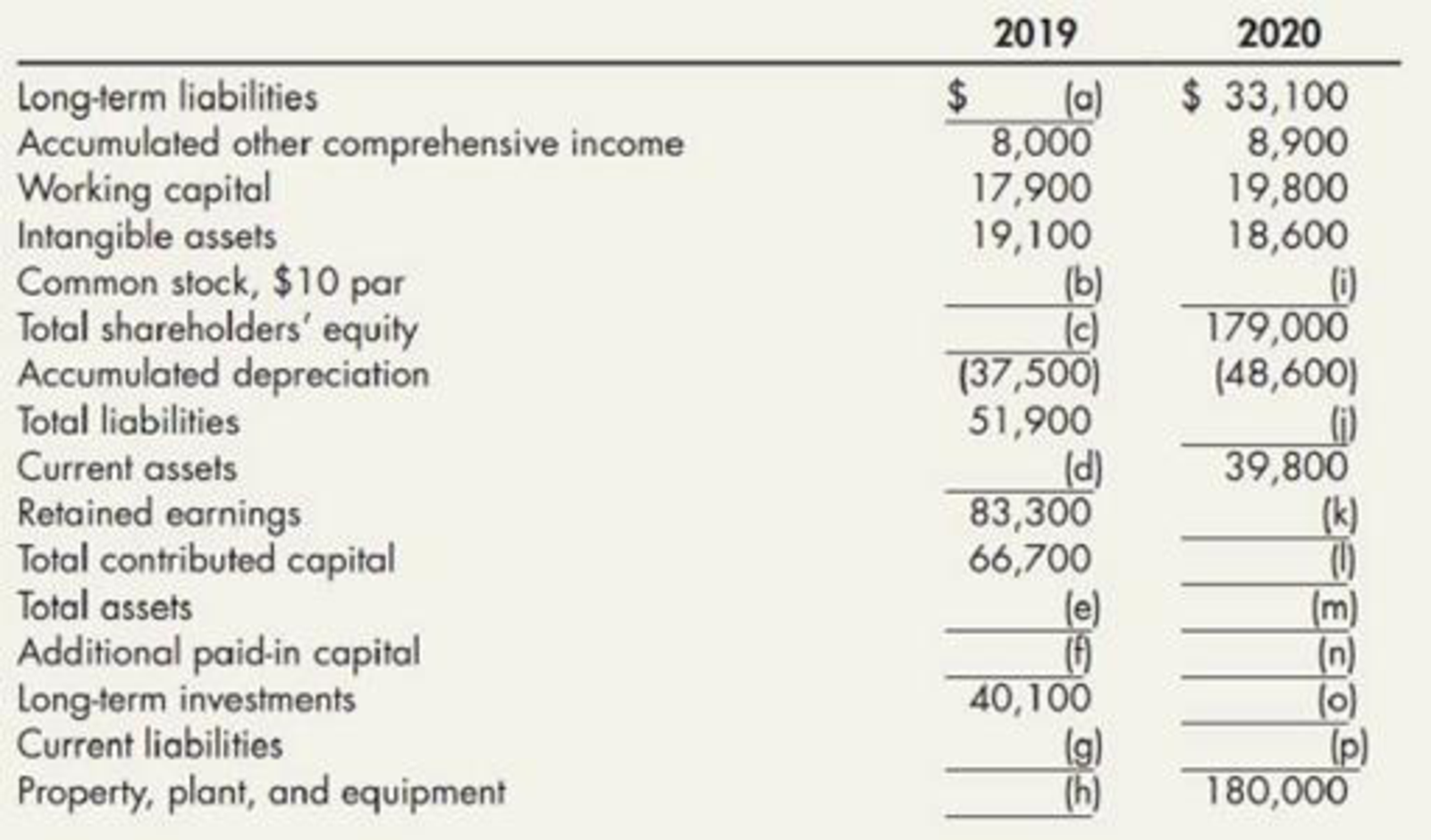Chapter 4, Problem 5P### Intermediate Accounting: Reporting...

3rd Edition
James M. Wahlen + 2 others
ISBN: 9781337788281

#### Solutions

Chapter
Section### Intermediate Accounting: Reporting...

3rd Edition
James M. Wahlen + 2 others
ISBN: 9781337788281
Textbook Problem
82 views

# Balance Sheet Calculations Cornerstone Development Company’s balance sheet information at the end of 2019 and 2020 is provided in random order, as follows:Additional information: At the end of 2019, (a) the amount of long-term liabilities is twice the amount of current liabilities and (b) there are 2,900 shares of common stock outstanding. During 2020, the company (a) issued 100 shares of common stock for $25 per share, (b) earned net income of$20,600, and (c) paid dividends of $1 per share on the common stock outstanding at year-end.Required:Next Level Fill in the blanks lettered (a) through (p). All of the necessary information is provided. (Hint: It is not necessary to calculate your answers in alphabetical order.) To determine Fill in the blanks labeled (a) through (p). Explanation Balance sheet: This financial statement reports a company’s resources (assets) and claims of creditors (liabilities) and stockholders (stockholders’ equity) over those resources. The resources of the company are assets which include money contributed by stockholders and creditors. Hence, the main elements of the balance sheet are assets, liabilities, and stockholders’ equity. Fill the missing information:  Company C Balance Sheet December 31 Particulars 2019 2020 Current assets (1)(d)$35,200 $39,800 Long-term investments$40,100 (8)(o)$42,300 Property, plant, and equipment (net) (2)(h)$153,000 $180,000 Less: Accumulated depreciation ($37,500) ($48,600) Intangible assets$19,100 $18,600 Total assets (e)$209,900 (m)$232,100 Current liabilities (3)(g)$17,300 (9)(p)$20,000 Long-term liabilities (4)(a)$34,600 $33,100 Total liabilities$51,900 (10)(j)$53,100 Common stock,$10 par (5)(b)$29,000 (11)(i)$30,000 Additional paid-in capital (6)(f)$37,700 (12)(n)$39,200 Total contributed capital $66,700 (13)(l)$69,200 Retained earnings $83,300 (14)(k)$100,900 Accumulated other comprehensive income $8,000$8,900 Total shareholders’ equity (7)(c)$158,000$179,000 Total liabilities and shareholders’ equity $209,900$232,100

Table (1)

Note:

The amount of total assets for missing information (e) for the year 2019 and (m) for the year 2020 is same as the amount of total liabilities and shareholders’ equity for the year 2019 ($209,900) and year 2020 ($232,100) since, total assets equals total of liabilities and shareholders’ equity.

Working notes:

Year 2019:

(1) Calculate the amount of current assets:

CurrentAssets=Currentliabilities+Workingcapital=$17,300+$17,900=$35,200 (2) Calculate the amount of property, plant and equipment: Property,plantandequipment=(TotalassetsCurrentassetsLong-terminvestmentsIntangibleassets+Accumulated depreciation)=$209,900$35,200$40,100$19,100+$37,500=$153,300 (3) Calculate the amount of current liabilities: Currentliability (x)=Total liabiltiyLong-termliability(2x)x=$51,9002x$51,900=2xx$51,900= -3x

Currentliability(x)=$51,9003=$51,9003=$17,300 (4) Calculate the amount of long-term liabilities: Long-termliabilities=Currentliabilities×2x=$17,300(3)×2xx=$34,600 (5) Calculate the amount of common stock: Coomonstock=Numberofsharesoutstanding×Parvalueofstock=2,900shares×$10=$29,000 (6) Calculate the amount of additional paid-in capital: Additonalpaid-incapital=TotalcontributedcapitalCommonsstock=$66,700$29,000(5)=$37,700

(7) Calculate the amount of total shareholders’ equity:

### Still sussing out bartleby?

Check out a sample textbook solution.

See a sample solution

#### The Solution to Your Study Problems

Bartleby provides explanations to thousands of textbook problems written by our experts, many with advanced degrees!

Get Started

#### What is inflation and what causes it?

Brief Principles of Macroeconomics (MindTap Course List)

#### What are problems associated with each one?

Foundations of Business (MindTap Course List)

#### BOND VALUATION An investor has two bonds in his portfolio that have a face value of 1,000 and pay a 10% annual ...

Fundamentals of Financial Management, Concise Edition (with Thomson ONE - Business School Edition, 1 term (6 months) Printed Access Card) (MindTap Course List)